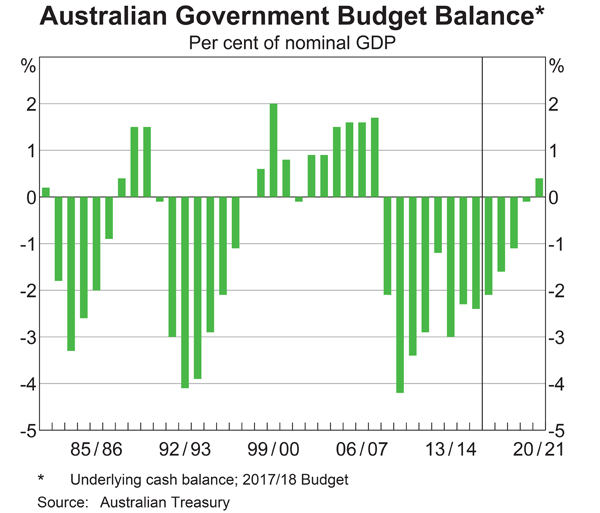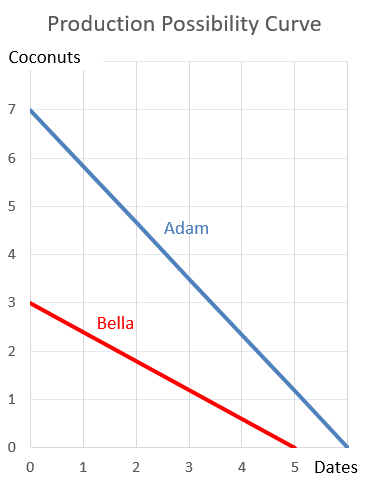Fight Finance

CoursesTagsRandomAllRecentScores

A stock is expected to pay the following dividends:

 Cash Flows of a Stock Time (yrs) 0 1 2 3 4 ... Dividend ($) 2 2 2 10 3 ... After year 4, the dividend will grow in perpetuity at 4% pa. The required return on the stock is 10% pa. Both the growth rate and required return are given as effective annual rates. What will be the price of the stock in 5 years (t = 5), just after the dividend at that time has been paid? The following is the Dividend Discount Model (DDM) used to price stocks: $$P_0=\dfrac{C_1}{r-g}$$ If the assumptions of the DDM hold, which one of the following statements is NOT correct? The long term expected: The following equation is the Dividend Discount Model, also known as the 'Gordon Growth Model' or the 'Perpetuity with growth' equation. $$p_0= \frac{c_1}{r-g}$$ Which expression is equal to the expected dividend return? Which of the following investable assets are NOT suitable for valuation using PE multiples techniques? Fred owns some Commonwealth Bank (CBA) shares. He has calculated CBA’s monthly returns for each month in the past 20 years using this formula: $$r_\text{t monthly}=\ln⁡ \left( \dfrac{P_t}{P_{t-1}} \right)$$ He then took the arithmetic average and found it to be 1% per month using this formula: $$\bar{r}_\text{monthly}= \dfrac{ \displaystyle\sum\limits_{t=1}^T{\left( r_\text{t monthly} \right)} }{T} =0.01=1\% \text{ per month}$$ He also found the standard deviation of these monthly returns which was 5% per month: $$\sigma_\text{monthly} = \dfrac{ \displaystyle\sum\limits_{t=1}^T{\left( \left( r_\text{t monthly} - \bar{r}_\text{monthly} \right)^2 \right)} }{T} =0.05=5\%\text{ per month}$$ Which of the below statements about Fred’s CBA shares is NOT correct? Assume that the past historical average return is the true population average of future expected returns. The phone company Optus have 2 mobile service plans on offer which both have the same amount of phone call, text message and internet data credit. Both plans have a contract length of 24 months and the monthly cost is payable in advance. The only difference between the two plans is that one is a: • 'Bring Your Own' (BYO) mobile service plan, costing$80 per month. There is no phone included in this plan. The other plan is a:
• 'Bundled' mobile service plan that comes with the latest smart phone, costing $100 per month. This plan includes the latest smart phone. Neither plan has any additional payments at the start or end. Assume that the discount rate is 1% per month given as an effective monthly rate. The only difference between the plans is the phone, so what is the implied cost of the phone as a present value? Given that the latest smart phone actually costs$600 to purchase outright from another retailer, should you commit to the BYO plan or the bundled plan?

Which of the following quantities from the Black-Scholes-Merton option pricing formula gives the Delta of a European put option?

You intend to use futures on oil to hedge the risk of purchasing oil. There is no cross-hedging risk. Oil pays no dividends but it’s costly to store. Which of the following statements about basis risk in this scenario is NOT correct?

Below is the Australian federal government’s budget balance as a percent of GDP.From 2009 to 2016 the Australian federal government has implemented:

Adam and Bella are the only people on a remote island. Their production possibility curves are shown in the graph.Which of the following statements is NOT correct?